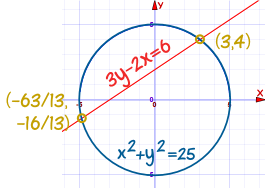# Systems of Linear and Quadratic EquationsA Linear Equation is an equation of a line.A Quadratic Equation is the equation of a parabola and has at least one variable squared (such as x2)And together they form a System of a Linear and a Quadratic Equation

A System of those two equations can be solved (find where they intersect), either:

• Graphically (by plotting them both on the Function Grapher and zooming in)
• or using Algebra

## How to Solve using Algebra

• Make both equations into "y =" format
• Set them equal to each other
• Simplify into "= 0" format (like a standard Quadratic Equation)
• Solve the Quadratic Equation!
• Use the linear equation to calculate matching "y" values, so we get (x,y) points as answers

An example will help:

### Example: Solve these two equations:

• y = x2 - 5x + 7
• y = 2x + 1

Make both equations into "y=" format:

They are both in "y=" format, so go straight to next step

Set them equal to each other

x2 - 5x + 7 = 2x + 1

Simplify into "= 0" format (like a standard Quadratic Equation)

Subtract 2x from both sides: x2 - 7x + 7 = 1
Subtract 1 from both sides: x2 - 7x + 6 = 0

Solve the Quadratic Equation!

(The hardest part for me)

You can read how to solve Quadratic Equations, but here we will factor the Quadratic Equation:

Start with: x2 - 7x + 6 = 0
Rewrite -7x as -x-6x: x2 - x - 6x + 6 = 0
Then: x(x-1) - 6(x-1) = 0
Then: (x-1)(x-6) = 0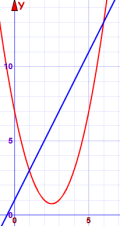Which gives us the solutions x=1 and x=6

Use the linear equation to calculate matching "y" values, so we get (x,y) points as answers

The matching y values are (also see Graph):

• for x=1: y = 2x+1 = 3
• for x=6: y = 2x+1 = 13

Our solution: the two points are (1,3) and (6,13)

I think of it as three stages:

Combine into Quadratic Equation ⇒ Solve the Quadratic ⇒ Calculate the points

## Solutions

There are three possible cases:

• No real solution (happens when they never intersect)
• One real solution (when the straight line just touches the quadratic)
• Two real solutions (like the example above)Time for another example!

### Example: Solve these two equations:

• y - x2 = 7 - 5x
• 4y - 8x = -21

Make both equations into "y=" format:

First equation is: y - x2 = 7 - 5x

Add x2 to both sides: y = x2 + 7 - 5x

Second equation is: 4y - 8x = -21

Add 8x to both sides: 4y = 8x - 21
Divide all by 4: y = 2x - 5.25

Set them equal to each other

x2 - 5x + 7 = 2x - 5.25

Simplify into "= 0" format (like a standard Quadratic Equation)

Subtract 2x from both sides: x2 - 7x + 7 = -5.25
Add 5.25 to both sides: x2 - 7x + 12.25 = 0

Solve the Quadratic Equation!

Using the Quadratic Formula from Quadratic Equations: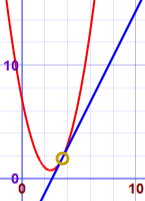• x = [ -b ± √(b2-4ac) ] / 2a
• x = [ 7 ± √((-7)2-4×1×12.25) ] / 2×1
• x = [ 7 ± √(49-49) ] / 2
• x = [ 7 ± √0 ] / 2
• x = 3.5

Just one solution! (The "discriminant" is 0)

Use the linear equation to calculate matching "y" values, so we get (x,y) points as answers

The matching y value is:

• for x=3.5: y = 2x-5.25 = 1.75

Our solution: (3.5,1.75)

### Real World Example

Kaboom!

The cannon ball flies through the air, following a parabola: y = 2 + 0.12x - 0.002x2

The land slopes upward: y = 0.15x

Where does the cannon ball land?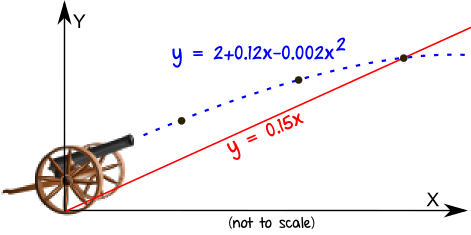Both equations are already in the "y =" format, so set them equal to each other:

0.15x = 2 + 0.12x - 0.002x2

Simplify into "= 0" format:

Bring all terms to left: 0.002x2 + 0.15x - 0.12x - 2 = 0
Simplify: 0.002x2 + 0.03x - 2 = 0
Multiply by 500: x2 + 15x - 1000 = 0

Solve the Quadratic Equation:

Split 15x into -25x+40x: x2 -25x + 40x - 1000 = 0
Then: x(x-25) + 40(x-25) = 0
Then: (x+40)(x-25) = 0
x = -40 or 25

The negative answer can be ignored, so x = 25

Use the linear equation to calculate matching "y" value:

y = 0.15 x 25 = 3.75

So the cannonball impacts the slope at (25, 3.75)

You can also find the answer graphically by using the Function Grapher: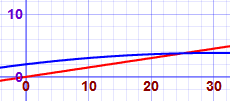.

## Both Variables Squared

Sometimes BOTH terms of the quadratic can be squared:

### Example: Find the points of intersection of

The circle x2 + y2 = 25

And the straight line 3y - 2x = 6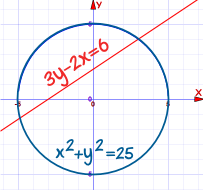First put the line in "y=" format:

Move 2x to right hand side: 3y = 2x + 6
Divide by 3: y = 2x/3 + 2

NOW, Instead of making the circle into "y=" format, we can use substitution (replace "y" in the quadratic with the linear expression):

Put y = 2x/3 + 2 into circle equation: x2 + (2x/3 + 2)2 = 25
Expand: x2 + 4x2/9 + 2(2x/3)(2) + 22 = 25
Multiply all by 9: 9x2 + 4x2 + 2(2x)(2)(3) + (9)(22) = (9)(25)
Simplify: 13x2+ 24x + 36 = 225
Subtract 225 from both sides: 13x2+ 24x - 189 = 0

Now it is in standard Quadratic form, let's solve it:

13x2+ 24x - 189 = 0
Split 24x into 63x-39x: 13x2+ 63x - 39x - 189 = 0
Then: x(13x + 63) - 3(13x + 63) = 0
Then: (x - 3)(13x + 63) = 0
So: x = 3 or -63/13

Now work out y-values:

Substitute x = 3 into linear equation:
• 3y - 6 = 6
• 3y = 12
• y = 4
• So one point is (3, 4)
Substitute x = -63/13 into linear equation:
• 3y + 126/13 = 6
• y + 42/13 = 2
• y = 2 - 42/13 = 26/13 - 42/13 = -16/13
• So the other point is (-63/13, -16/13)